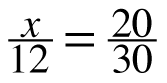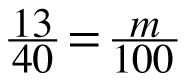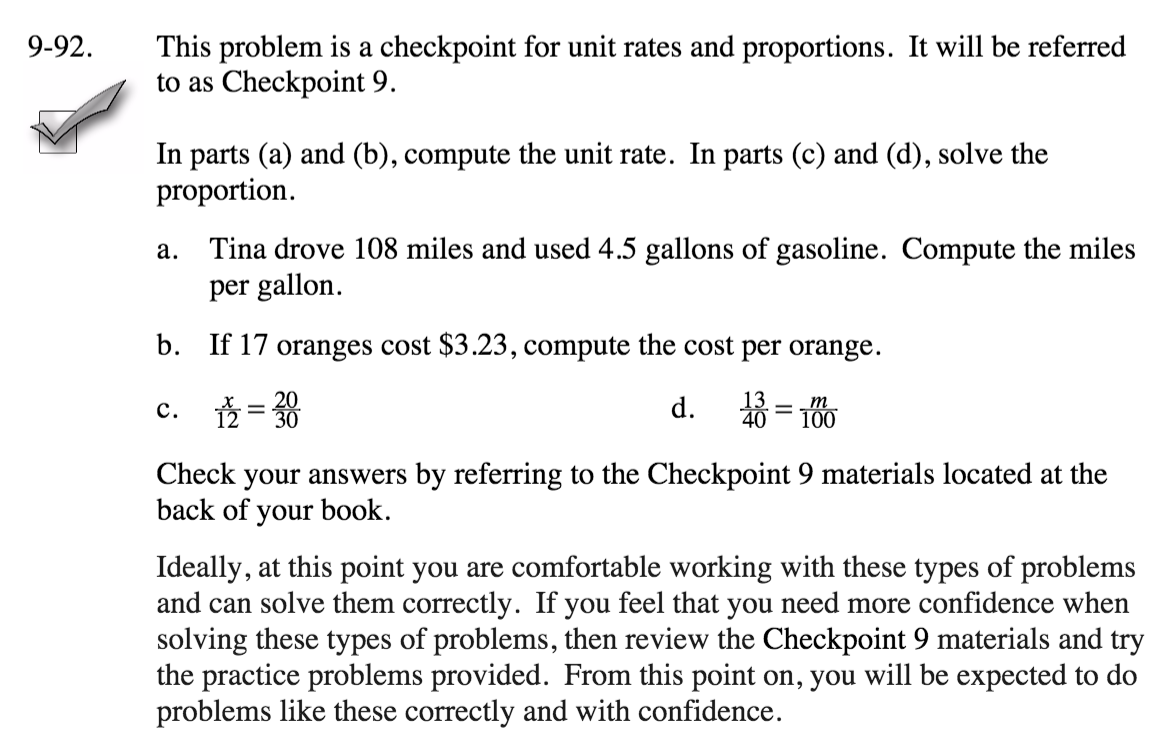Home > CC2 > Chapter 9 > Lesson 9.2.4 > Problem9-92

9-92.
1. This problem is a checkpoint for unit rates and proportions. It will be referred to as Checkpoint 9.2. In parts (a) and (b), compute the unit rate. In parts (c) and (d), solve the proportion. Homework Help ✎

1. Tina drove 108 miles and used 4.5 gallons of gasoline. Compute the miles per gallon.

2. If 17 oranges cost \$3.23, compute the cost per orange.

3.4.3. Check your answers by referring to the Checkpoint 9 materials.

4. Ideally, at this point you are comfortable working with these types of problems and can solve them correctly. If you feel that you need more confidence when solving these types of problems, then review the Checkpoint 9 materials and try the practice problems provided. From this point on, you will be expected to do problems like these correctly and with confidence.Answers and extra practice for the Checkpoint problems are located in the back of your printed textbook or in the Reference Tab of your eBook. If you have an eBook for CC2, login and then click the following link: Checkpoint 9: Unit Rates and Proportions